#### You may also like### Consecutive Numbers

An investigation involving adding and subtracting sets of consecutive numbers. Lots to find out, lots to explore.### Calendar Capers

Choose any three by three square of dates on a calendar page...### Days and Dates

Investigate how you can work out what day of the week your birthday will be on next year, and the year after...

# Linear Area

##### Age 11 to 14 ShortChallenge Level

Sketch diagram
$y=-2$ means that the $y$ coordinate will always be $-2$, regardless of what $x$ is. So this is the line through $(3,-2)$, $(-6,-2)$, $(0,-2)$ and $(1000000,-2)$ - that is, a horizontal line 'along' $-2$: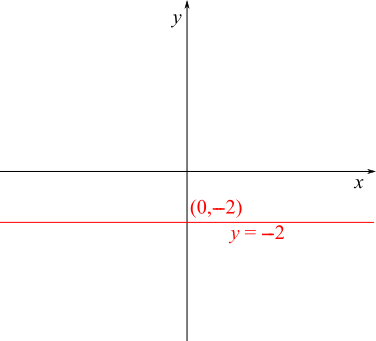Next, $y=4x+6$ has gradient $4$ and $y$-intercept $6$ - so it slopes up steeply and crosses the $y$ axis at $(0,6)$.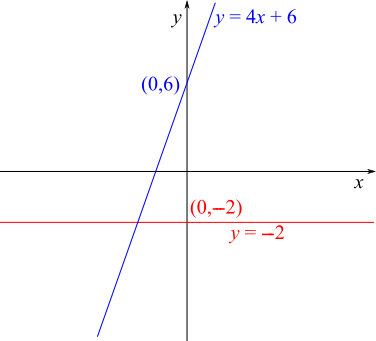Next, $x+y=6$ has gradient $-1$ and $y$ intercept $6$ ($x+y=6$ is the same as $y=-x+6$). So it slopes downwards, much more gently than the blue line, and also crosses the $y$ axis at $(0,6)$: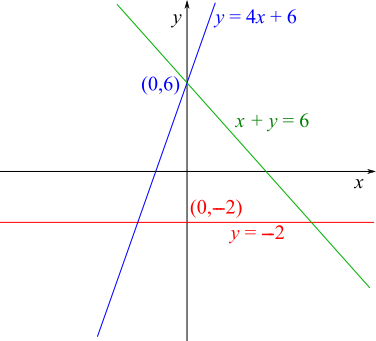You can already see that the height of the triangle is $8$ units, from $-2$ to $6$ on the $y$ axis.

For the base of the triangle...
Finding the coordinates of the points of intersection
Suppose $y=-2$ and $y=4x+6$ intersect at some point $(a,b)$.
$(a,b)$ is on the line $y=-2$, so $b=-2$.
$(a,b)$ is on the line $y=4x+6$, so $b=4a+6$. But $b=-2$, so $-2=4a+6\Rightarrow -8=4a\Rightarrow -2=a$.

So $y=-2$ and $y=4x+6$ intersect at $(-2,-2)$.

Suppose $y=-2$ and $x+y=6$ intersect at some point $(c,d)$.
$(c,d)$ is on the line $y=-2$, so $d=-2$.
$(c,d)$ is on the line $x+y=6$, so $c+d=6$. But $d=-2$, so $c-2=6\Rightarrow c=8$.

So $y=-2$ and $x+y=6$ intersect at $(8,-2)$. This is shown below.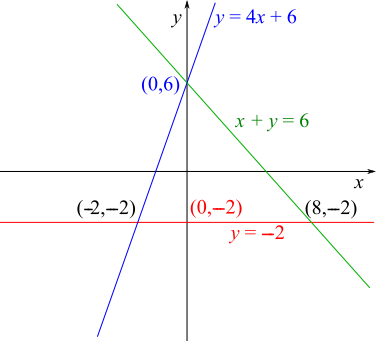So the base of the triangle is from $x=-2$ to $x=8$, which is $10$ units.

So the area of the triangle is $\frac12\times8\times10=40$ square units.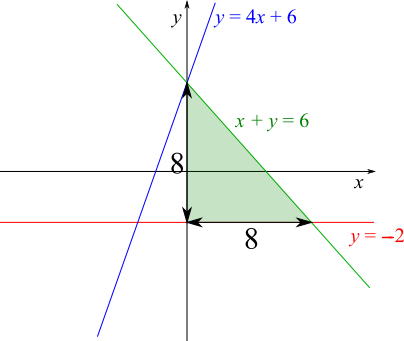The gradient of the line $x+y=6$ is $-1$, so when going down $8$ units from the top to the bottom of the triangle, it must also go along $8$ units, as shown on the right.

The line $y=4x+6$ has gradient $4$, so it is $4$ times steeper. This means that going down by the same amount corresponds to going $4$ times less far along.

So the base of the blue triangle shown below is only $8\div4=2$ units.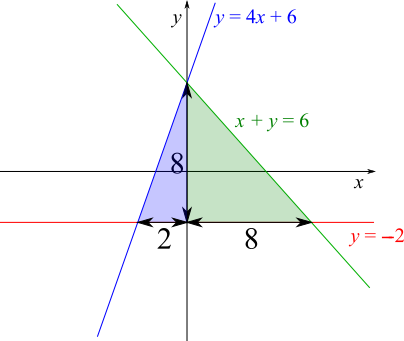We could find the areas of the green and blue triangles separately, or of the whole triangle, whose base is $10$ units in total.

Green triangle: $\frac12\times8\times8=32$ square units

Blue triangle: $\frac12\times8\times2=8$ square units

Whole triangle: $32+8=40$ square units, or $\frac12\times8\times10=40$ square units.

Plotting the lines on a grid
Plotting the lines carefully, you can find the dimensions of the triangle.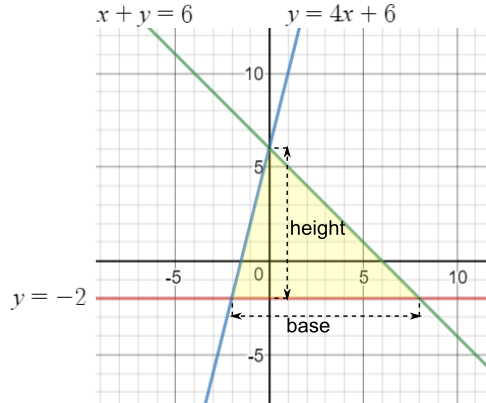Now, you can count that the base is $10$ units and the height is $8$ units, so the area is $\frac12\times8\times10=40$ square units.
You can find more short problems, arranged by curriculum topic, in our short problems collection.